# MISSION BANKING 2023 Quantitative Aptitude Quiz- 21

## MISSION BANKING 2023 Quantitative Aptitude Quiz

Quantitative Aptitude is the most significant part of almost all competitive exams. Candidates appear to be having difficulty with Quantitative Aptitude Questions, so they need to practice more. Even if the portion is difficult, if you practice enough questions and learn the basic concepts, you can surely do well. In this article, we have come up with the MISSION BANKING 2023 Quantitative Aptitude Quiz to enhance your preparation. This MISSION BANKING 2023 Quantitative Aptitude Quiz will assist you to cope with the tough competition. This MISSION BANKING 2023 Quantitative Aptitude Quiz is available to you at no cost. Through this MISSION BANKING 2023 Quantitative Aptitude Quiz, you will learn formulas and short tricks to solve the Quantitative Aptitude Questions. Candidates must solve this MISSION BANKING 2023 Quantitative Aptitude Quiz to improve their exam.

Directions (1-5): In each of these questions, two equations (I) and (II) are given. You have to solve both the equations and give answer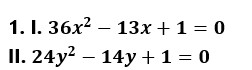(a) if x>y

(b)  if x≥y

(c) if x<y

(d) if x ≤y

(e) if x = y or no relation can be established between x and y.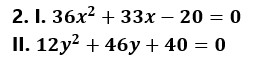(a) if x>y

(b)  if x≥y

(c) if x<y

(d) if x ≤y

(e) if x = y or no relation can be established between x and y.(a) if x>y

(b)  if x≥y

(c) if x<y

(d) if x ≤y

(e) if x = y or no relation can be established between x and y.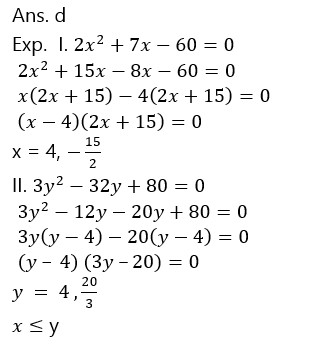(a) if x>y

(b)  if x≥y

(c) if x<y

(d) if x ≤y

(e) if x = y or no relation can be established between x and y.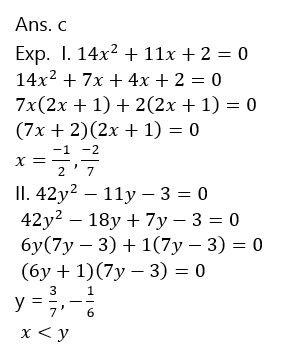(a) if x>y

(b)  if x≥y

(c) if x<y

(d) if x ≤y

(e) if x = y or no relation can be established between x and y.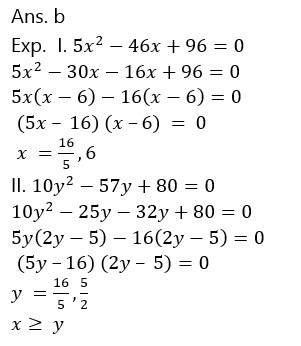1. A, B and C independently can do a work in 15 days, 20 days, and 30 days respectively. They work together for some days after which C leaves and remaining work is completed by A and B together. Total Rs. 18000 is paid for the work and ‘B’ gets Rs. 6000 more than ‘C’. For how many days did ‘A’ worked?

(a) 8 Days

(b) 4 Days

(c) 6 Days

(d) 10 Days

(e) 2 Days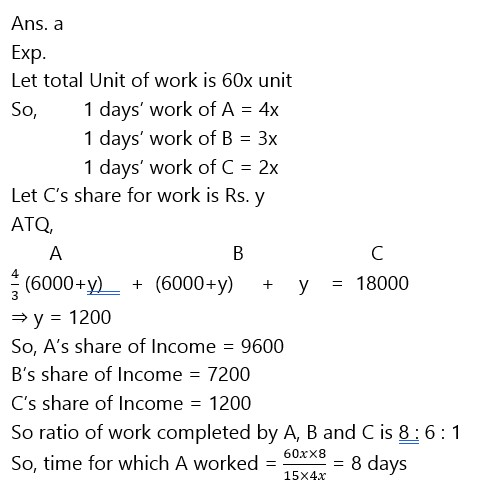1. A train ‘X’ travelling at a speed of 50 kmph crosses another train ‘Y’ coming from opposite direction at a speed of 40 kmph in 12 sec. Length of train ‘Y’ is 50% less than that of train ‘X’. If train ‘X’ passes a railway platform in 45 seconds then find the length of the platform?

(a) 425 m

(b) 400 m

(c) 620 m

(d) 360 m

(e) 500 m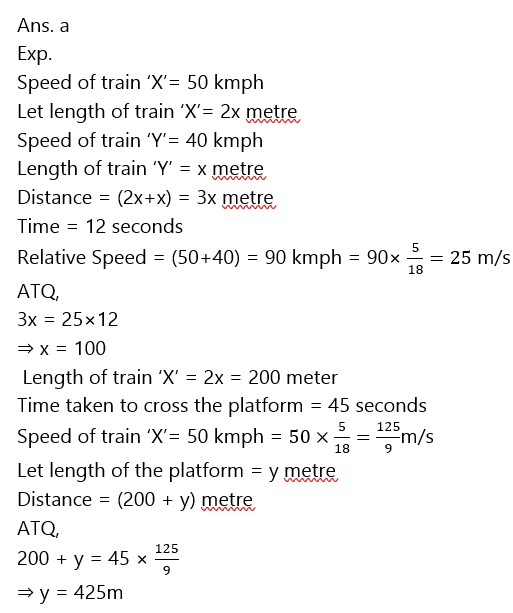1. The respective ratio of milk and water in the mixture is 4 : 3 respective. If 6 litre of water is added to this mixture, the respective ratio of milk and water becomes 8 : 7. Find the quantity of milk in the original mixture?

(a) 96 litre

(b) 36 litre

(c) 84 litre

(d) 48 litre

(e) 24 litre1. Ratio of speed of boat in still water to speed of stream is 5 : 1. Boat goes from point A to B (120 km) upstream and return back to A in total 13.5 hours, but during return journey, speed of boat reduces by 20%. Find the speed of stream?

(a) 4 kmph

(b) 3kmph

(c) 2 kmph

(d) 1 kmph

(e) 6 kmph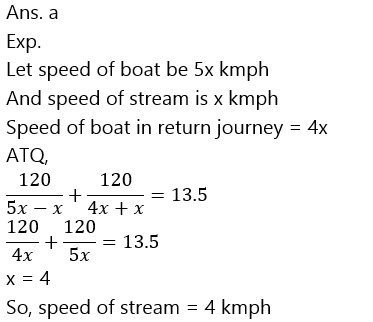1. If the ratio between compound interest received in first year and in only second year is 5 : 6 and Interest earned in only third year is Rs. 720 more than interest earned in only second year then find the principal amount.

(a) Rs. 9250

(b) Rs. 13500

(c) Rs. 15000

(d) Rs. 25000

(e) Rs. 22800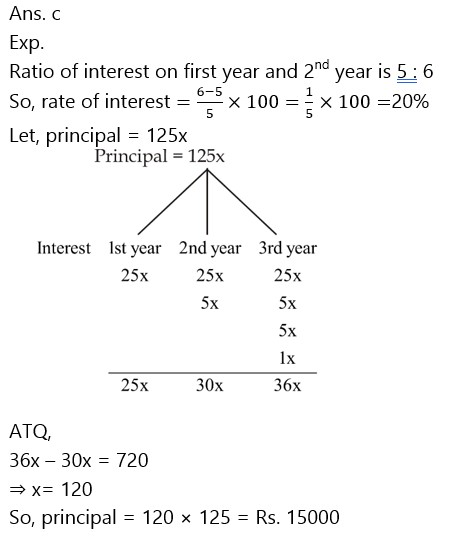### 2023 Preparation Kit PDF

#### Most important PDF’s for Bank, SSC, Railway and Other Government Exam : Download PDF Now

AATMA-NIRBHAR Series- Static GK/Awareness Practice Ebook PDF Get PDF here
The Banking Awareness 500 MCQs E-book| Bilingual (Hindi + English) Get PDF here
AATMA-NIRBHAR Series- Banking Awareness Practice Ebook PDF Get PDF here
Computer Awareness Capsule 2.O Get PDF here
AATMA-NIRBHAR Series Quantitative Aptitude Topic-Wise PDF 2020 Get PDF here
Memory Based Puzzle E-book | 2016-19 Exams Covered Get PDF here
Caselet Data Interpretation 200 Questions Get PDF here
Puzzle & Seating Arrangement E-Book for BANK PO MAINS (Vol-1) Get PDF here
ARITHMETIC DATA INTERPRETATION 2.O E-book Get PDF here

3